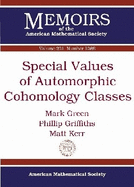## Special Values Of Automorphic Cohomology Classes

The authors study the complex geometry and coherent cohomology of nonclassical Mumford-Tate domains and their quotients by discrete groups. Their focus throughout is on the domains $D$ which occur as open $G(\mathbb{R})$-orbits in the flag varieties for $G=SU(2,1)$ and $Sp(4)$, regarded as classifying spaces for Hodge structures of weight three. In the context provided by these basic examples, the authors formulate and illustrate the general method by which correspondence spaces $\mathcal{W}$ give rise to Penrose transforms between the cohomologies $H^{q}(D,L)$ of distinct such orbits with coefficients in homogeneous line bundles.

• Condition: --
• Format: Paperback
• Sold by: --
• Language: English
• Publisher: Amer Mathematical Society
• ISBN-13: 9780821898574
• ISBN: 0821898574
• Publication Year: 2014
Loading...
Loading marketplace...
×